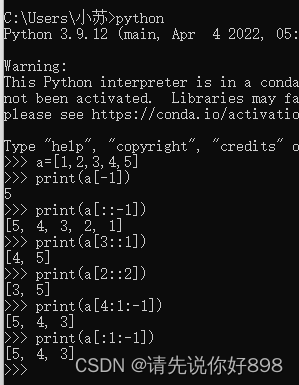# python：数组取值

a=[1,2,3,4,5]；

a=1；a=2；a=3；a=4；a=5;

(1) a[-1]：b=print(a[-1])=print(取出数组a的最后一个值)=;

(2) a[:-1]：b=print(a[:-1])=print(除了最后一个取全部)=[1,2,3,4];

因为[m:n]在数组里边表示的是前闭后开，就相当于数学里边的[m:n)，取不到最后一个索引值;

(3) a[1:]：b=print(a[1:])=print(从索引为1的位置开始向最后一位取值，当结束索引处无固定值时就取到最后一个值)=[2,3,4,5];

(4) [::-1]： b=print([::-1])=print(从最后一位向前取值)=[5，4，3，2，1];代表从最后一位向前取值，从全列表倒序，从后向前一次取值；跟上边的二维不一样的是当中间结束索引为空时代表取至最后一个值，也就没有所谓的前闭后开了；反之如果中间的索引有值时就要进行前闭后开的规则；

a[3::1]    b=print(a[3::1])=print(从索引为3开始取到最后，步长为1)=[4，5]；

a[3::-1]    b=print(a[3::-1])=print(从索引为3开始取到最前边一个，步长为1)=[4，3，2，1]；

a[2::2]    b=print(a[2::2])=print(从索引为2位置开始向最后一位取值，步长为2)=[3，5];

a[4:1:-1]=a[:1:-1]    b=print(a[4:21-1])=print(从索引为4的开始逆向取值至索引为1的值)=[5,4,3];（其中索引为2的值取不到);

因为它可以是[n : m : k]的具体化，代表的是从n开始取，m是结束取值的索引（此时也是该索引对应的值取不到，只能取m-1索引对应的值），隔k个取一次（该k值可以取正可以取负，正代表的是正向取值（n<m)，负代表反向取值(n>m));

np.random.rand(d0,d1,d2……dn)：通过本函数可以返回一个或一组服从“0~1”均匀分布的随机样本值。随机样本取值范围是[0,1)，不包括1；

np.random.randn()内部输入值为整数，若为浮点数则自动直接截取并转换为相应的整数值输入,作用就是通过np.random.randn()可以返回一个或一组服从标准正态分布的随机样本值（值可大于1）；
np.random.standard_normal（）的输入参数为元组（tuple）;

（1）np.random.rand(3,2)：表示生成一个三行两列的矩阵（值都是0-1之间的小数）；

（2）np.random.rand(2,3,4)：表示生成一个二维的三行四列的矩阵；（值都是0-1之间的）；

（3）其中a[:,:,0]：表示输出矩阵中二维中的第0个索引的所有元素；